Wang, X., Zhou, A., and Chui, Y. (2018). "Load-carrying capacity of intermediately slender parallel strand bamboo columns with a rectangular cross section under biaxial eccentric compression," BioRes. 13(1), 313-330.

#### Abstract

Parallel strand bamboo (PSB), a processed bamboo composite, has been used as an alternative to wood products in building and engineering applications. Inelastic analysis of PSB members is important because of the evident nonlinearity of the material. The present work conducted experimental investigations on intermediately slender PSB columns subjected to biaxial eccentric loads. The bending failure, which underwent a pronounced nonlinear process, was the major characteristic of the intermediately slender PSB column that had a biaxial compressive load. The mid-height cross section of the columns can be divided into three parts over its depth: the plastic compressive zone, the elastic compressive zone, and the tensile zone. Both the secondary bending and material nonlinearity were important factors that impacted the nonlinear response of the columns. An analytical method was presented for evaluating the load-carrying capacity of intermediately slender PSB columns with rectangular cross sections under biaxial eccentric compression loads. There was good agreement between the results of the experiments and calculations.

Load-carrying Capacity of Intermediately Slender Parallel Strand Bamboo Columns with a Rectangular Cross Section under Biaxial Eccentric Compression

Xiaorui Wang,a,b Aiping Zhou,a,* and Ying Hei Chui c

Parallel strand bamboo (PSB), a processed bamboo composite, has been used as an alternative to wood products in building and engineering applications. Inelastic analysis of PSB members is important because of the evident nonlinearity of the material. The present work conducted experimental investigations on intermediately slender PSB columns subjected to biaxial eccentric loads. The bending failure, which underwent a pronounced nonlinear process, was the major characteristic of the intermediately slender PSB column that had a biaxial compressive load. The mid-height cross section of the columns can be divided into three parts over its depth: the plastic compressive zone, the elastic compressive zone, and the tensile zone. Both the secondary bending and material nonlinearity were important factors that impacted the nonlinear response of the columns. An analytical method was presented for evaluating the load-carrying capacity of intermediately slender PSB columns with rectangular cross sections under biaxial eccentric compression loads. There was good agreement between the results of the experiments and calculations.

Contact information: a: School of Civil Engineering, Nanjing Forestry University, 159 Longpan Road, Nanjing, China, 210037; b: Faculty of Forestry and Environmental Management, University of New Brunswick, Fredericton, New Brunswick, Canada, E3B 5A3; c: Department of Civil and Environmental Engineering, University of Alberta, 116 St. and 85 Ave., Edmonton, AB, Canada, T6G 2R3;

* Corresponding author: zaping2007@163.com

INTRODUCTION

Bamboo has been widely used in the construction industry as a sustainable building material because of its excellent mechanical performance, its fast growth, and its environmentally friendly features (Amada and Untao 2001; Zhou et al. 2012). However, raw bamboo cannot meet the requirements of modern buildings because of the diversity in the mechanical properties and dimensions. Parallel strand bamboo (PSB) is a processed bamboo composite that is fabricated by gluing parallel bamboo strands together under a controlled temperature and pressure (Huang et al. 2015b, 2016). Because bamboo strips are sorted according to their age and growing conditions, PSB has controllable properties and dimensions and are suitable for use as a construction material.

Columns are a common structural member that can carry combined bending and compression loads. They are often treated as an eccentrically compressed member in design calculations. The slenderness can remarkably affect the load-carrying capacity of a column. The load-carrying capacity of a stocky column depends on the material properties, while the strength of a slender column depends on the slenderness ratio (Timoshenko and Gere 1961). For an intermediately slender column, whose slenderness ratio falls between that of stocky and slender, the load-carrying capacity is dominated by both its slenderness and material properties. Analytical models for the load-carrying capacity calculation of intermediately slender steel and concrete columns subjected to eccentric compression have been well studied and widely accepted in design codes of concrete and steel structures (Chen and Atsuta 1977; ANSI/AISC 360-10 2010; ACI 318M-14 2015). However, the analytical models for steel and concrete calculations cannot be used for intermediately slender PSB column calculations because of the complicated constitutive laws of the material. These laws have been confirmed by Huang et al. (2013), who reported that the parallel-to-grain constitutive law of PSB exhibits linearity in tension, whereas it exhibits pronounced nonlinearity in compression, which leads to remarkable nonlinearity in PSB bending members. The same effect impacts the combined bending and compression PSB members, which are discussed later in this paper.

Lots of studies on timber columns have been done. Buchanan et al. (1985) suggested a design method for timber engineering. Yoshihara (2010) analyzed the elastic buckling of plywood columns. Streiger and Fontana (2005) and Theiler et al. (2013) put forward their respective calculation models for solid timber columns under bending moments and axial forces. An ultimate-state-based method to predict the load-carrying capacity of PSB columns under uniaxial eccentric compression has been proposed by Huang et al. (2015a). However, there have been few studies on either wood or bamboo composite columns subjected to biaxial eccentric loads. The theoretical calculation of biaxially loaded columns is more complex than for uniaxially loaded columns. Most current design methods for timber structures, which are based on the allowable stress design (ASD) or load and resistance factor design (LRFD) concepts, provide normal force-moment interaction equations as the failure criterion to determine whether the member is safe (EN 1995-1-1 2004; ASD/LRFD 2005; GB 50005-2003 2005; O86-09 2009; CWC 2010). These equations cannot be used to predict the load-carrying capacity of columns.

This study investigated the failure mode and damage mechanism of intermediately slender PSB columns under biaxial eccentric compression loads. An analytical method to compute the load-carrying capacity of these columns was proposed. The calculation procedure used a successive approximation method to avoid the difficulties of iterative computations of a problem, which included both the material properties and geometric nonlinearity. The model predictions, test results, and design codes were compared.

EXPERIMENTAL

Material Properties

Bamboo culms measuring 2000 mm in length were split into bamboo filament bundles by passing through a roll crusher. Phenolic glue was used to manufacture the parallel bamboo strand lumber specimens under a pressure of 4 MPa at 160 °C. The final moisture content of PSB specimens was 9.11%, and the density was 1.08 g/cm3. Before the column experiments, the mechanical properties of PSB were tested according to ASTM D143-14 (2014). Because the column analysis only involves the parallel-to-strand properties of PSB composites, the test only studied the parallel-to-strand compression and tension performance. Figure 1 schematically illustrates the stress-strain relationship in the parallel-to-strand direction of the PSB composite, and the associated parameters are presented in Table 1. The empirical constitutive equation for a PSB composite, created by Huang et al. (2015b), is shown in Eq. 1,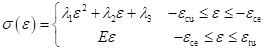(1)

where, σcu and εcu are the compressive strength and ultimate compressive strain, respectively, σce and εce are the stress and strain corresponding to the proportional compressive limit, respectively, σtu and εtu are the ultimate tensile strength and strain, respectively, and E is the longitudinal Young’s modulus. The constants λi (i = 1, 2, 3) can be obtained by data fitting.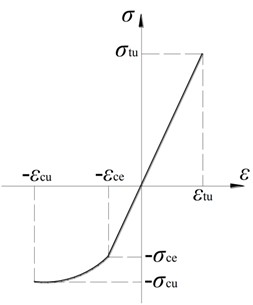Fig. 1. Longitudinal stress–strain relationship of the PSB

Table 1. Longitudinal Mechanical Parameters of the PSB Composite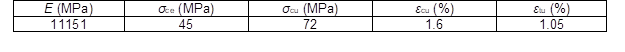Test Procedure

The damage mechanism and failure mode of intermediately slender columns loaded with biaxial eccentric compression loads was investigated. According to ASTM D143-14 (2014) for stocky columns and Euler’s theory on the global stability of columns, the slenderness ratio of test specimens was between 17 and.

Three groups of columns with the same cross section (100 mm × 100 mm) and different slenderness ratios were tested. The slenderness ratios and column lengths of each group are presented in Table 2. The parameters of eccentricity (e) and the skew angle (β) on which the load was applied are schematically shown in Fig. 2. Because the test columns were pinned at both ends, the slenderness ratio was calculated by Eq. 2. The skew angle was determined with Eq. 3,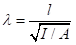(2)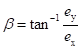(3)

where ex and ey are the eccentricities in the X and Y-directions, respectively, and A and I are the area and moment of inertia of the section, respectively.

Table 2. Dimensions and Eccentricities of the Tested Columns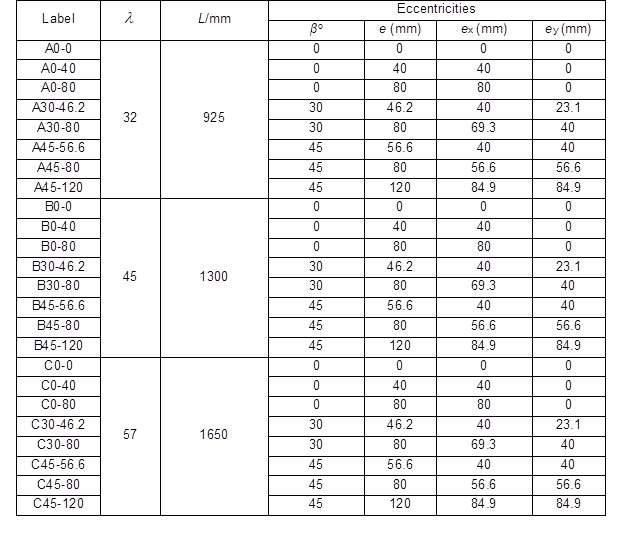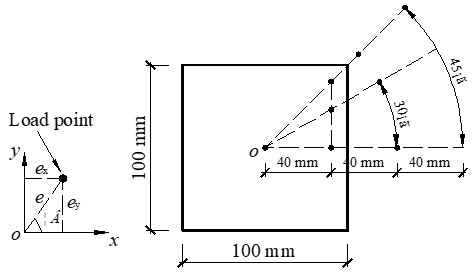Fig. 2. Load points at the ends of the columns

Figure 3a illustrates the uniaxially loaded columns. Five longitudinally orientated and equidistant strain gauges were glued on a side surface at the mid-height of the test samples. The lateral displacement at the mid-height was measured by a horizontally mounted deformation sensor. For the biaxially loaded columns (Fig. 3b), the longitudinal strains at the mid-height cross section were measured by four strain gauges, which were glued at the corner of each side surface. The lateral displacement in two principal directions at the mid-height were monitored by two deformation sensors.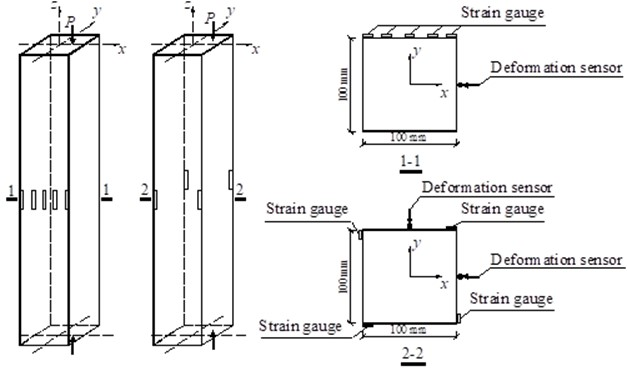(a)                                    (b)                                                  (c)

Fig. 3. Instrumentation for the strain gauges and deformation sensors: a) uniaxial compression, b) biaxial compression, and c) mid-span cross section information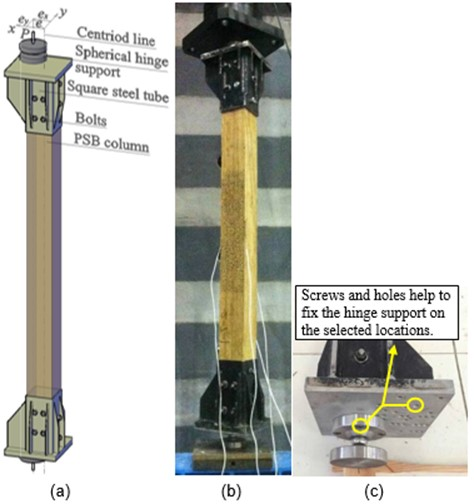Fig. 4. Test setup: a) schematic, b) test setup, and c) details

Compressive forces were applied to the test samples by two steel boots mounted at each end of the column. Two spherical bearings were placed between the boots and machine supporter or actuator to enable free rotation at each end. The test specimens were vertically installed in the test machine in such a manner that the applied force coincided with the line through the centroid of the two spherical bearings and parallel to the undeformed column. The distances between the centroid line and load line were the initial eccentricities of the load. Hence, any desired eccentricity was achieved by adjusting the position of the spherical bearings, as is shown in Fig. 4. The load was monotonically applied at a constant speed of 2 mm/min until the specimen failed. The load, lateral deflection, and strain of each gage were recorded simultaneously at a frequency of 1 Hz.

RESULTS AND DISCUSSION

Figure 5 shows the typical failure modes of the tested columns. The fibers at the remote corner or surface in the convex side were broken in tension when failure took place. A major crack consequently originated from the broken area and rapidly expanded along the longitudinal direction at the moment that the fiber broke. The cracks went through the two adjacent or opposite surfaces of the broken corner. The failure mode of these columns was similar to the bending failure of a beam. Figures 5a, 5b, and 5c demonstrate three typical failure modes that occurred for different load eccentricities. The failures suggested that the orientation of the crack surfaces depended on the load eccentricities. Also, the normal direction of the crack surface approximately coincided with the line going through the cross sectional centroid and the action point of the load. This implied that bending took place in the plane through the line from the centroid of the cross section and load point, and the warping of the column was trivial.

Figure 6 shows the typical load vs. the mid-span lateral displacement curves for the specimens with different slenderness ratios and load eccentricities. It was evident that the specimens exhibited significant inelastic before failure. The ultimate load-carrying capacity of the columns decreases with the increasing of the slenderness ratios and load eccentricities. The ultimate lateral displacement at the mid-span can reach approximately 3% of the column length.

Figure 7a shows the longitudinal strains over the mid-span section of the column labeled B0-80. The section was divided into three parts over its depth: the plastic compressive zone (PCZ), in which the fiber strains all exceeded the elastic proportional limit (εce); the elastic compressive zone (ECZ), in which the fiber strains were less than the elastic proportional limit; and the tensile zone (TZ), in which the fibers were in a tensile state. At the five measured points, the strain was approximately linear from the beginning of loading to failure. This demonstrated that the plane assumption, i.e., plane sections remain plane after deformation, was nearly correct for these PSB columns. Furthermore, Fig. 7b shows the local details on an enlarged scale. The neutral axis of the mid-span section was stationary in the elastic stage, and it moved approximately 4 mm towards the tensile side during the plastic process. This result indicated that the fibers in the concave side were progressively compressed into a nonlinear stress state from the surface to the inside of the compressive zone.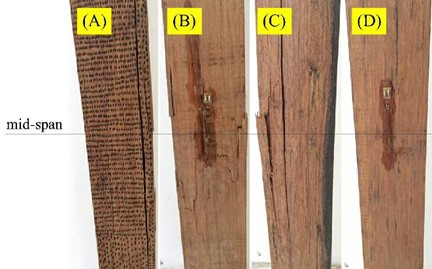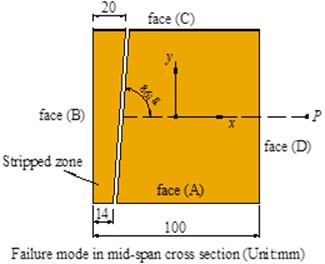(a)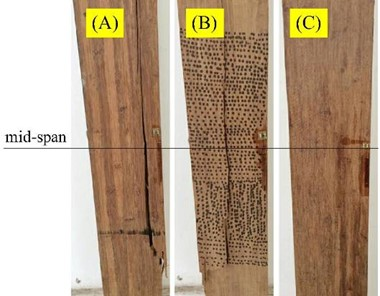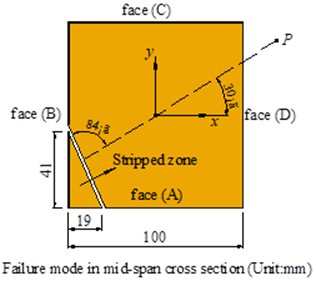(b)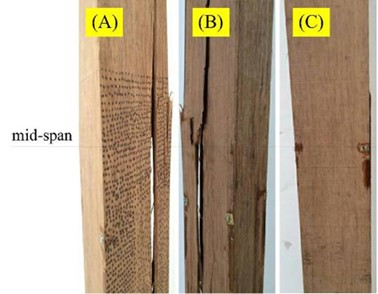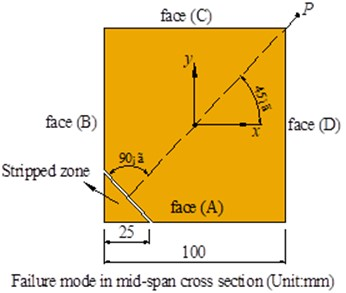(c)

Fig. 5. Typical failure modes of the columns for β values of 0° (a), 30° (b), and 45° (c)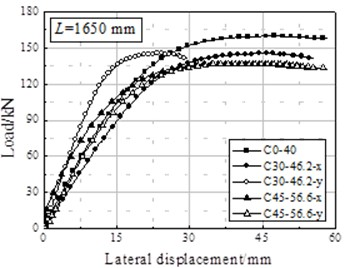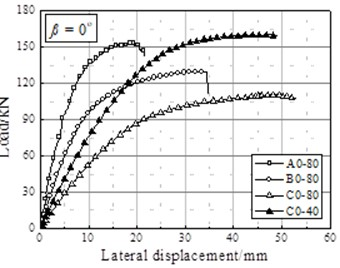(a)                                                                                                       (b)

Fig. 6. Comparisons of the load-lateral displacement curves for different columns: a) eccentricity effect, and b) slenderness effect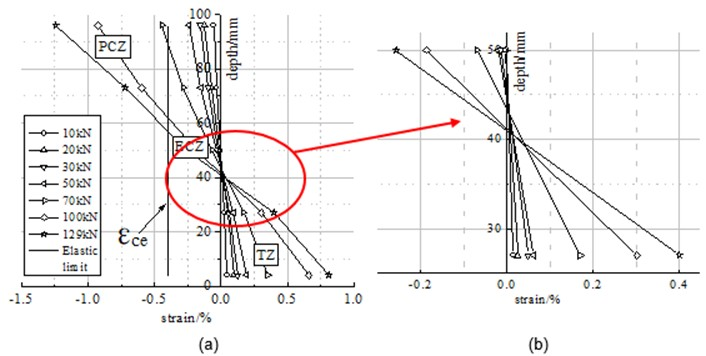Fig. 7. Mid-span cross section strains of the uniaxially-loaded columns: a) B0-80, and b) details

Figures 8a and 8b show the development of strains at each corner of the columns A30-80 and A45-80, respectively. Point B represents the fiber strain at the corner on the concave side, and point D represents the fiber strain at the corner on the convex side. The nonlinear process started with the outermost compressive strains reaching the elastic proportional limit (εce = 0.4%). Thus, the compressive strain at the corner nearest to the position of loading reached the yielding limit first, and the yielding area progressively extended to the inner area of the section. Similar to the uniaxially loaded columns, the damaged section was also divided into three zones, i.e., PCZ, ECZ, and TZ. The strain at the remote corner of the section reached the ultimate value when failure occurred.

Because the warping can be neglected in the rectangular cross section column (Chen and Atsuta 1977; Iwai et al. 1986), the plane assumption was still correct for the biaxially loaded PSB columns. Based on the plane assumption and the strains at each corner of the columns in Figs. 8a and 8b, the strain distribution over the mid-span cross section was obtained. Using the A30-80 and A45-80 columns as examples, the deformations of the mid-span cross section of these two columns are shown in Figs. 8c and 8d, respectively. The line EeFe was the neutral axis in the elastic state, and the line EuFu was the neutral axis in the ultimate state. The separatrix GH defined the boundary between the PCZ and ECZ in the ultimate state. The neutral line and separatrix were parallel to each other and formed a trapezoidal TZ in the A30-80 column and a triangular TZ in the A45-80 column. Additionally, the neutral axis in the ultimate state was basically parallel to that in the elastic state and shifted in parallel towards the tensile corner during loading. This was consistent with the conclusions from Fig. 7.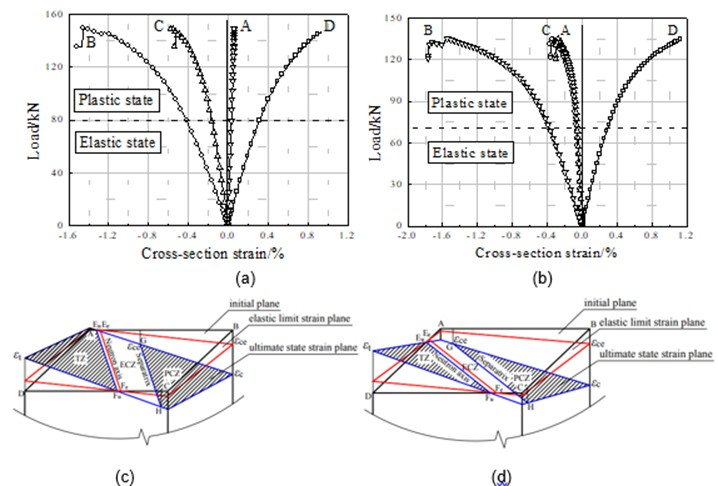Fig. 8. Mid-span cross section strain distribution of the biaxially loaded columns: a) A30-80, b) A45-80, c) A30-80, and d) A45-80

In summary, the bending failure, which exhibited a pronounced nonlinear process, was the major failure mode of the intermediately slender PSB column with a biaxial compression load.

The peak load appeared when the outer most fiber strains reached the material ultimate strain. The plane assumption was verified. The neutral axis moved in parallel to the tensile side during the loading process.

Modeling of the Columns

According to the failure modes and the strain analysis of the failure cross section, the following hypotheses were adopted to develop a model for the nonlinear analysis of the biaxially loaded PSB columns: (1) the plane remains in plane during bending; (2) shearing and warping can be neglected; and (3) the neutral axis of bending in the damaged cross section always keeps its original orientation during loading.

Calculation of the Lateral Displacement of the Mid-Span Cross Section

Figure 9 illustrates a pin-supported column symmetrically loaded with eccentric compression loads at its two ends. Cartesian coordinates, which were originated from the centroid of the cross section with X- and Y-axes parallel to the two principal directions, were employed for the sake of creating modeling equations. The Z-axis was in the longitudinal direction of the undeformed column. The variables ex0 and ey0 stood for the initial eccentricities of the thrust (P).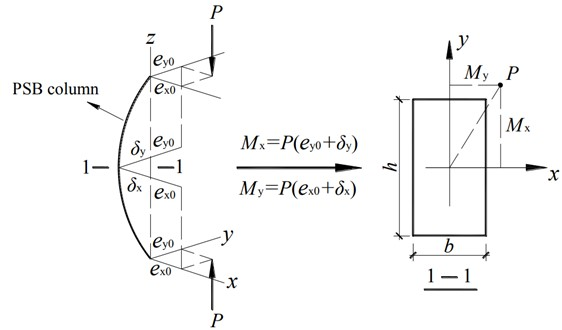Fig. 9. Columns subjected to biaxial eccentric compression

The effect of the slenderness on the second order bending of the column was taken into consideration with the Moment Magnification Method (Engesser 1895). The variables δx and δydenoted the lateral deflections in the two principal directions, and the moment magnification factor (μ) was expressed with the following equation,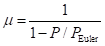(4)

where PEuler is the plastic Euler buckling load of the column, l is the effective length, I is the moment of inertia of the section, and Et is the tangent modulus of the material proposed by Engesser (1895), which is the instantaneous slope of the stress-strain curve at the point corresponding to the buckling stress. The Et value is approximately 0.8E for timber, and the effectiveness of this reduction of the modulus of elasticity in timber columns was verified by Steiger and Fontana (2005). In terms of biaxial bending, the moment magnification factors were computed for each axis; thus, the deflections in each principal direction were computed with Eqs. 5 and 6.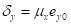,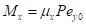(5)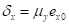,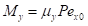(6)

Analysis of the Cross Section

As discussed above, the damaged cross section consisted of 3 zones, i.e., TZ, ECZ, and PCZ. The possible configurations of the cross section are schematically demonstrated in Fig. 10. In case 1 and 3, the neutral axis cuts the two opposite boundaries of the cross section and forms a trapezoidal compressive zone. In case 2, the neutral axis intersects the adjacent boundaries, forming a triangular compressive zone. For a square cross section, case 1 and 3 become the same situation. As such, only two cases needed to be considered during modeling.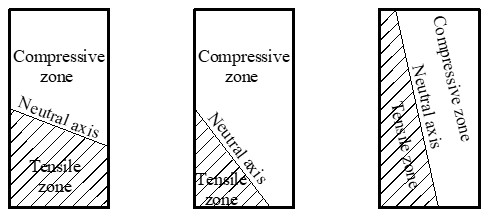Fig. 10. Possible positions of the neutral axis

A trapezoidal TZ was used as the example on how to estimate the load-carrying capacity of the column. Figure 11 illustrates a damaged rectangular section with a trapezoidal TZ. The inclination of the neutral axis is θ, and the depths of TZ, ECZ, and PCZ are ytyce, and ycu, respectively.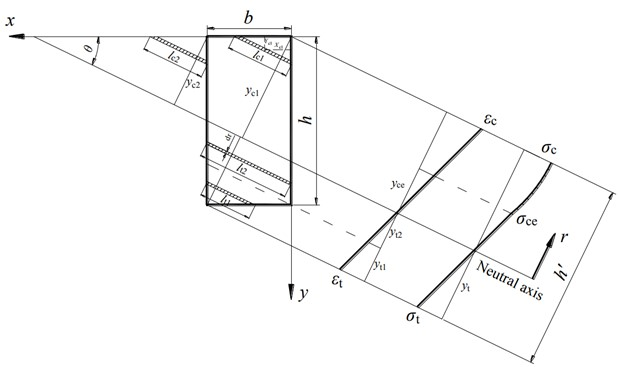Fig. 11. Typical behavior of a rectangular cross section under biaxially-loaded compression

It was assumed that the bending curvatures of the column around the two principal axes, X and Y, are ϕx and ϕy, and thus the strain at any point (xy) on the cross section was expressed as follows,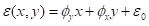(7)

where ε0 is the strain at the centroid of the cross section (b/2, h/2), which can be calculated by Eq. 8.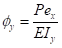,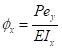,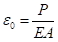(8)

The neutral axis of the biaxial bending cross section was expressed as Eq. 9.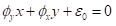(9)

The neutral axis is a line in the cross section, and the angle of inclination of the neutral axis was computed by Eq. 10.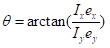(10)

As discussed, the orientation of the neutral axis kept its original direction during the entire loading process. In the ultimate state, the relationship between the depths of the TZ (yt) and ECZ (yce) was expressed by Eq. 11.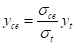(11)

The applied forces, NMx, and My, were obtained by integrating the stress over the cross section. However, the complicated integration and high order equations made it impossible to obtain explicit expressions for the resultant forces calculation. Hence, this paper employed the superposition method, which was verified by Chen and Santathadaporn (1969), to calculate the load-carrying capacity. For example, for yce less than yc2, the internal force was calculated as follows,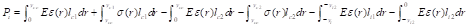(12)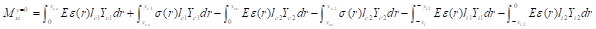(13)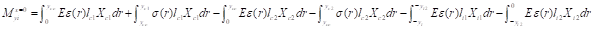(14)

where,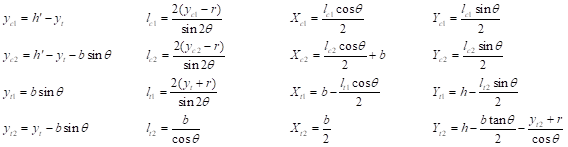(15)

The meaning of each variable can be found in Fig. 11; they are obtained by geometric relationships. The solution was obtained by iterative calculation, in which the depth of the TZ (yt) was successively changed until Pi was sufficiently close to P.

Calculation Procedure

A procedure was developed to calculate the ultimate load-carrying capacity of an intermediately slender column under a compressive load with eccentricities (ex0 and ey0). A flowchart of the successive approximation procedure based on the aforementioned method is illustrated in Fig. 12. The calculation started by selecting an initial force (P0) and the height of the TZ (yt). The magnified mid-span moments (Mx and My) and the internal resultant load and moments (PiMxi, and Myi) were computed. The values of P0 and yt were successively updated until the equilibrium equations were satisfied. The accuracy of this procedure was controlled by the increments in each step and the target error (Δ).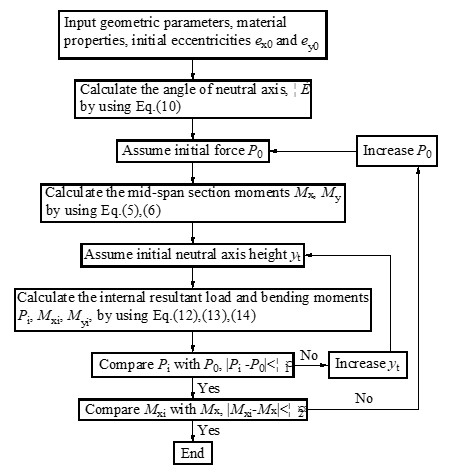Fig. 12. Calculation flow chart

The calculated results were compared with the experimental values (Table 3). N is the ultimate load obtained by the tests, and Nu is the predicted capacity. The load-carrying capacities calculated by the proposed model agreed fairly well with the results obtained from the experiments, with a few exceptions. These exceptions may have been because of the variability in the material properties and the mean values of the material properties were used in the calculations.

Figure 13 shows the comparison of the M-N interaction diagram among the predictions of the proposed model, test results, and design codes for PSB columns with a 1300 mm length under uniaxial eccentric compression. There was no design code that could predict the ultimate state of the mid-span cross section of the PSB columns. The CSA/AWC methods and the stability equation of Eurocode 5 may be used in checking the end-span cross section. According to Eurocode 5, the stress equation squares the compression part in the interaction model, but does not take probable buckling into consideration (EN 1995-1-1 2004).

Table 3. Comparison of the Load-carrying Capacities from the Proposed Model Predictions and Experiment Results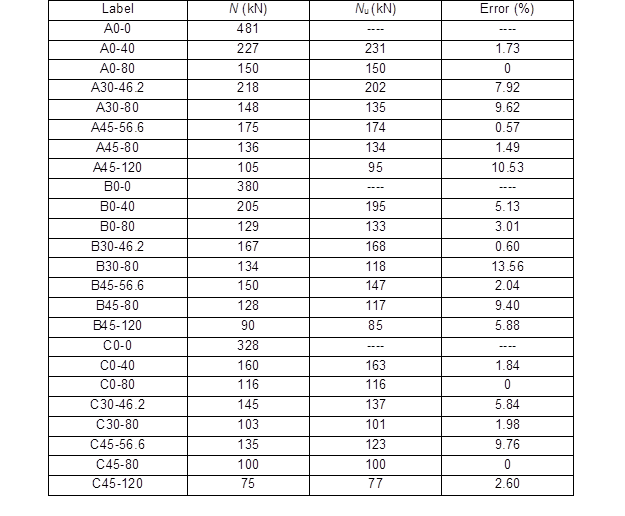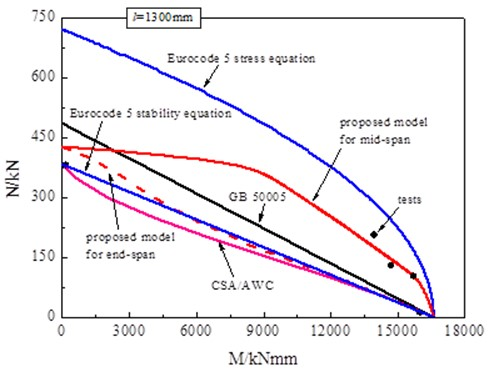Fig. 13. Comparison of the proposed model predictions, test results, and design codes

The stability equation uses a buckling factor to reduce the compressive strength, and simply suggests a linear interaction between the bending moments and axial force. The separation of the stability factor and stress factor in a column led to a relatively conservative result. However, the M-N envelope of the proposed model can fit the test results well because this method took both the material properties and geometric nonlinearity into consideration.

CONCLUSIONS

1. Bending failure is the major characteristic of intermediately slender PSB columns loaded with a biaxial compressive load. The peak load appeared when the outermost fiber strains reached the material ultimate strain.
2. The damage of the columns underwent a pronounced nonlinear process before failure. Both the secondary bending and material nonlinearity were important factors that impacted the nonlinear response of the columns.
3. The mid-height cross section of columns can be divided into three parts over its depth: the plastic compressive zone (PCZ), the elastic compressive zone (ECZ), and the tensile zone (TZ). The neutral axis between ECZ and TZ moved in parallel to the tensile side during loading process.
4. Based on the experimental study, an analytical method, which took both the material properties and geometric nonlinearities into account, was presented for evaluating the load-carrying capacity of intermediately slender PSB columns with rectangular cross sections under biaxial eccentric compression. Good agreements were achieved between the results of the experiments and calculations.

ACKNOWLEDGMENTS

The authors are grateful for the support of the National Science Fund of China (No. 51578291), National Twelfth-five Scientific Support Plan of China (No. 2015BAL03B02), Priority Academic Program Development of Jiangsu Higher Education Institutions, and the Doctorate Fellowship Foundation of Nanjing Forestry University.

REFERENCES CITED

ACI 318M-14 (2015). “Metric building code requirements for structural concrete and commentary,” American Concrete Institute, Farmington Hills, MI.

Amada, S., and Untao, S. (2001). “Fracture properties of bamboo,” Compos. Part B-Eng. 32(5), 451-459. DOI: 10.1016/S1359-8368(01)00022-1

ANSI/AISC 360-10 (2010). “Specification for structural steel buildings,” American Institute of Steel Construction, Chicago, IL.

ASD/LRFD (2005). Manual for Engineered Wood Construction, American Forest & Paper Association, American Wood Council, Washington, DC.

ASTM D143-14 (2014). “Standard test methods for small clear specimens of timber,” ASTM International, West Conshohocken, PA.

ASTM D198-09 (2009). “Standard test methods of static test of lumber in structural sizes,” ASTM International, West Conshohocken, PA.

Buchanan, A. H., Johns, K. C., and Madsen, B. (1985). “Column design methods for timber engineering,” Can. J. Civil Eng. 12(4), 731-744. DOI: 10.1139/I85-088

CWC (2010). Wood Design Manual 2010, Canadian Wood Council (CWC), Ottawa, Canada.

Chen, W. F., and Atsuta, T. (1977). Theory of Beam-Columns Volume 2: Space Behavior and Design, McGraw-Hill, New York, NY.

Chen, W. F., and Santathadaporn, S. (1969). “Curvature and the solution of eccentrically loaded columns,” J. Eng. Mech. Div.-ASCE 95(1), 21-40.

EN 1995-1-1 (2004). “Eurocode 5: Design of timber structures – Part 1-1: General – Common rules and rules for buildings,” European Committee for Standardization, Brussels, Belgium.

Engesser, F. (1895). “Buckling,” Schweizerische Bauzeitung 25(13), 88-90.

GB 50005-2003 (2005). “Code for design of timber structures,” Standardization Administration of China, Beijing, China.

Huang, D., Bian, Y., Huang, D., Zhou, A., and Sheng, B. (2015a). “An ultimate-state-based-model for inelastic analysis of intermediate slenderness PSB columns under eccentrically compressive load,” Constr. Build. Mater. 94, 306-314. DOI: 10.1016/j.conbuildmat.2015.06.059

Huang, D., Bian, Y., Zhou, A., and Sheng, B. (2015b). “Experimental study on stress-strain relationships and failure mechanisms of parallel strand bamboo made from phyllostachys,” Constr. Build. Mater. 77, 130-138. DOI: 10.1016/j.conbuildmat.2014.12.012

Huang, D., Zhou, A., and Bian, Y. (2013). “Experimental and analytical study on the nonlinear bending of parallel strand bamboo beams,” Constr. Build. Mater. 44, 585-592. DOI: 10.1016/j.conbuildmat.2013.03.050

Huang, Z., Chen, Z., Huang, D., and Zhou, A. (2016). “The ultimate load-carrying capacity and deformation of laminated bamboo hollow decks: Experimental investigation and inelastic analysis,” Constr. Build. Mater. 117, 190-197. DOI: 10.1016/j.conbuildmat.2016.04.115

Iwai, S., Minami, K., and Wakabayashi, M. (1986). “Stability of slender reinforced concrete columns subjected to biaxially eccentric loads,” Bulletin of the Disaster Prevention Research Institute 36(3-4), 137-157.

Steiger, R., and Fontana, M. (2005). “Bending moment and axial force interacting on solid timber beams,” Mater. Struct. 38(5), 507-513. DOI: 10.1007/BF02479541

Theiler, M., Frangi, A., and Steiger, R. (2013). “Strain-based calculation model for centrically and eccentrically loaded timber columns,” Eng. Struct. 56, 1103-1116. DOI: 10.1016/j.engstruct.2013.06.032

Timoshenko, S. P., and Gere, J. M. (1961). Theory of Elastic Stability, McGraw-Hill, New York, NY.

Yoshihara, H. (2010). “Analysis of the elastic buckling of a plywood column,” Mater. Struct. 43(8), 1075-1083. DOI: 10.1617/s11527-009-9567-y

Zhou, A., Huang, D., Li, H., and Su, Y. (2012). “Hybrid approach to determine the mechanical parameters of fibers and matrixes of bamboo,” Constr. Build. Mater. 35, 191-196. DOI: 10.1016/j.conbuildmat.2012.03.011

Article submitted: September 6, 2017; Peer review completed: October 22, 2017; Revised version receive and accepted: November 3, 2017; Published: November 20, 2017.

DOI: 10.15376/biores.13.1.313-330